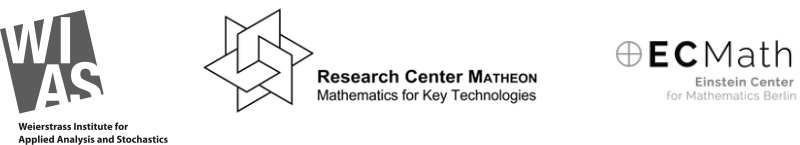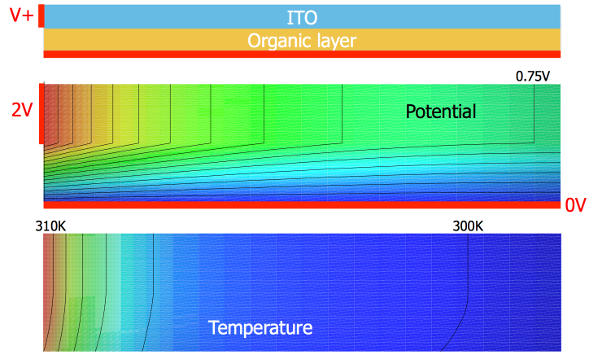## Project SE2: Electrothermal modeling of large-area OLEDs

#### This research is carried out in the framework of Matheon supported by Einstein Foundation Berlin

Project heads: Staff: Collaboration: External Cooperation: Internal Cooperation:

### Project background

Organic Light-Emitting Diodes (OLEDs) are thin-film multilayer devices based on organic molecules or polymers. These devices are not only used as displays, but they also occur in intelligent lighting applications. Despite their increasing use in commercial products many technical issues in the development of flat-panel OLED lightings, e.g. spatially non-uniform light emission due to self-heating, still exist. Therefore, accurate methods for modeling the electronic properties combined with the thermal management are needed to design efficient and temperature-tolerant device structures.

### Aim of project SE2

The aim of the Matheon project SE2 is to find adequate spatially resolved PDE models that describe the interplay between current and heat flow leading to self-heating and thermal switching phenomena in organic semiconductor devices. Moreover, the project intends to investigate their analytical properties, derive suitable numerical approximation schemes, and provide simulation results which can help to optimize large-area organic light emitting diodes.Schematic cross section of an OLED stack. (More)

### Thermistor modeling

For organic semiconductor devices the Arrhenius law applies: The electric conductivity increases with rising temperature. Therefore the electrical current increases and the device gets hotter, resulting in a feedback loop which continuously heats up the entire structure. Experiments show that this effect often leads to the destruction of the device if the heat cannot be dispersed into the environment, see Fischer et al. 2012. It was demonstrated in Fischer et al. 2013 that organic devices with small active areas can be described by a zero-dimensional thermistor model, where the isothermal current-voltage relation for the circuit is given by a power law and a temperature-dependent conductivity factor resulting from an Arrhenius law. Here, the crucial parameter is the so-called activation energy of the material that describes the disorder in the system. For sufficiently high activation energies the self-heating of the device results in S-shaped current-voltage characteristics with negative differential resistance resulting in hysteretic behavior and electric bistability.

### Spatially resolved PDE modeling

In Fischer et al. 2014, S-shaped IV curves were experimentally verified for OLED structures using 4-wire measurements. Due to the high sheet resistance of the optically transparent electrode and the corresponding lateral voltage drop, spatial dependence is introduced. In this case, the zero-dimensional thermistor has to be replaced by an array of electrically and thermally coupled thermistors to explain the observed formation of inhomogeneities in the luminance.

Based on these equivalent circuit models, in Liero et al. 2015 a stationary PDE thermistor model was derived, which is more flexible in view of numerical simulation and analytical treatment. It consists of the heat equation for the temperature coupled to the current-flow equation for the electrostatic potential with mixed boundary conditions. In particular, it features the same Arrhenius-like temperature law and non-Ohmic behavior of the organic layers. More details

### Analysis for p(x)-Laplace thermistor models

The existence of solutions for the p-Laplace thermistor problem for constant exponent p ≥ 2 in two dimensions was first shown in Liero et al. 2015. Known techniques for the treatment of the p(x)-Laplace terms fail in the setting of organic devices since they suppose a log-Hölder continuous dependency of p(x). Generalizations to variable discontinuous exponents p and higher spatial dimensions can be found in Glitzky-Liero 2015 and Bulíček-Glitzky-Liero 2015.

As a first approach, in Glitzky-Liero 2017 a discontinuous, piecewise constant exponent p(x) ≥ 2 was considered in two dimensions. A novel higher integrability results for the gradient of the electrostatic potential was proved by means of localization, Caccioppoli estimates, and Gehring-type arguments. The resulting higher integrability of the Joule heat term in the heat flow equation allows to prove the solvability of the p(x)-Laplace thermistor problem by Schauder’s fixed-point theorem.

A second approach is presented in Bulíček-Glitzky-Liero 2016a. Therein, higher spatial dimensions and arbitrary exponents p(x) are considered. The existence proof is based on a regularized version of the thermistor system, where the crucial Joule heat term is approximated such that it remains bounded. The existence of solutions to the regularized problem is proven by Galerkin approximations. By using suitable test functions for the regularized system, uniform estimates independent of the regularization parameter were derived, which allows to pass to the limit and to obtain at least one weak solution to the p(x)-Laplace thermistor problem. Finally, in Bulíček-Glitzky-Liero 2016b the notion of entropy solutions of the heat equation in conjunction with Schauder's fixed point theorem is investigated in the same setting.S-shaped current-voltage characteristics in organic devices. (More)

### Numerical schemes

A finite-volume approximation of the full PDE thermistor model was introduced in Liero et al. 2015, where an accurate implementation of the non-standard electrical conductivity law and the Joule heat expression in the case of non-Ohmic materials is included. Test cases for p(x)-Laplace-type problems with piecewise constant, but considerably jumping p show the stability and performance of the scheme. The most challenging task is the computation of the S-shaped IV curves. Following the ideas by J. Fuhrmann and K. Gärtner path-following techniques where included to overcome the intrinsic instabilities in the system and to compute complete S-shaped characteristics.

### Software

We are developing a software tool for the simulation of the interplay between current and heat flow in large-area OLEDs. In particular, we solve the thermistor problem detailed in Liero et al. 2015 in 1D, 2D, and 3D using finite-volume methods. This is based on the PDELib2 toolbox developed at the Weierstrass Institute.Solutions of p(x)-Laplace thermistor system in 2D. (More)

### Events

We organized the workshop "AMaSiS 2015: Applied Mathematics and Simulation for Semiconductors". The workshop took place from March 11-13, 2015 at WIAS Berlin.

In cooperation with ECMath project SE4, A. Glitzky and T. Koprucki coorganized of the Kick-off meeting for the ECMI Special Interest Group "Sustainable Energy" (December 8-9, 2014 at TU Berlin). In the associated workshop "Nanostructures for Photovoltaics and Energy Storage" a session on "Electrical and electrothermal modeling and simulation of organic materials and devices" was initiated.

### Contact

We are seeking for partners for industrial applications, for further application-oriented development of our models and software, and for joint applications for public grants.

### Publications

The following list shows our publications related to organic semiconductors and associated problems. They cover modeling aspects as well as related mathematical properties of models and numerical methods.

1. A PDE model for electrothermal feedback in organic semiconductor devices Matheon preprint 1112
2. Convergence to equilibrium in energy-reaction-diffusion systems using vector-valued functional inequalities WIAS preprint 2349
3. Thermistor systems of p(x)-Laplace-type with discontinuous exponents via entropy solutions to appear in DCDS-S, WIAS preprint 2247, 2016 Link: WIAS Preprint
4. Systems describing electrothermal effects with p(x)-Laplacian like structure for discontinuous variable exponents SIAM Journal on Mathematical Analysis, 48(5), 3496-3514, 2016
5. Operation mechanism of high performance organic permeable base transistors with an insulated and perforated base electrode J. Appl. Phys., 120, pp. 094501/1--094501/8, 2016
6. Modeling of edge-emitting lasers based on tensile strained germanium microstripes. IEEE Photonics Journal, 7:1502115/1–1502115/15, 2015
7. Robustness analysis of a device concept for edge-emitting lasers based on strained germanium Optical and Quantum Electronics, 48, pp. 156/1--156/7, 2016
8. Modeling of an edge-emitting strained-Ge laser In Advanced Solid State Lasers, Advanced Solid State Lasers, page ATu2A.19/1. Optical Society of America, 2015
9. OLEDs: light-emitting thin film thermistors revealing advanced self-heating effects Proc. SPIE, 9566, 95661A–95661A-7, 2015
10. On device concepts for CMOS-compatible edge-emitters based on strained germanium In J. Piprek and W. Yuh-Renn, editors, Proceedings of the 15th International Conference on Numerical Simulation of Optoelectronic Devices 2015, pages 129–130. IEEE Conference Publications Management Group, Piscataway, 2015
11. Analysis of p(x)-Laplace thermistor models describing the electrothermal behavior of organic semiconductor devices Nonlinear Analysis: Real World Applications, 34, pp. 536--562,2017 Link: WIAS Preprint
12. p-Laplace thermistor modeling of electrothermal feedback in organic semiconductors Zeitschrift für angewandte Mathematik und Physik, volume 66, number 6, pp. 2957–2977, 2015
13. On microscopic origins of generalized gradient structures Discrete Contin. Dyn. Syst. Ser. S, 10, pp. 1--35, 2017 Link: WIAS Preprint
14. Homogenization of Cahn-Hilliard-type equations via evolutionary Gamma-convergence WIAS preprint 2114, 2015 Link: WIAS Preprint
15. Convergence of an implicit Voronoi finite volume method for reaction-diffusion problems Numerical Methods for Partial Differential Equations NUMPDE, 32:141–174, 2016
16. Uniform global bounds for solutions of an implicit Voronoi finite volume method for reaction-diffusion problems Numer. Math., 128(1):31–72, 2014.
17. Uniform estimate of the relative free energy by the dissipation rate for finite volume discretized reaction-diffusion systems In Ch. Rohde J. Fuhrmann, M. Ohlberger, editor, Finite Volumes for Complex Applications VII – Methods and Theoretical Aspects, pages 275–283. vol. 77 of Springer Proceedings in Mathematics & Statistics, Springer, Heidelberg, 2014
18. Feel the heat: Nonlinear electrothermal feedback in Organic LEDs Advanced Functional Materials, Volume 24, Issue 22, pp. 3367–3374, 2014
19. Electro-thermal modeling of organic semiconductors describing negative differential resistance induced by self-heating Proceedings of the 13th International Conference on Numerical Simulation of Optoelectronic Devices, NUSOD 2013, 19–22 August, 2013
20. Self-heating, bistability, and thermal switching in organic semiconductors Phys. Rev. Lett., Volume 110, Issue 12, pp. 126601/1–126601/5, 2013
21. Self-heating effects in organic semiconductor crossbar structures with small active area Organic Electronics, Volume 13, Issue 11, pp. 2461–2468, 2012